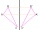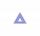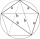# Pythagorean theorem - math word problems

#### Number of problems found: 704

• Cone containerRotary cone-shaped container has a volume 1000 cubic cm and a height 12 cm. Calculate how much metal we need for making this package.Calculate the surface area and volume of a regular quadrangular pyramid: sides of bases (bottom, top): a1 = 18 cm, a2 = 6cm angle α = 60 ° (Angle α is the angle between the side wall and the plane of the base.) S =? , V =?
• Calculate 8Calculate the coordinates of point B axially symmetrical with point A[-1, -3] along a straight line p : x + y - 2 = 0.
• Pile of sandA large pile of sand has been dumped into a conical pile in a warehouse. The slant height of the pile is 20 feet. The diameter of the base of the sand pile is 31 feet. Find the volume of the pile of sand.
• Hexagonal pyramidFind the volume of a regular hexagonal pyramid, the base edge of which is 12 cm long and the side edge 20 cm.
• Medians and sidesDetermine the size of a triangle KLM and the size of the medians in the triangle. K=(-5; -6), L=(7; -2), M=(5; 6).
• Five-gonCalculate the side a, the circumference and the area of the regular 5-angle if Rop = 6cm.
• The regularThe regular triangular prism has a base in the shape of an isosceles triangle with a base of 86 mm and 6.4 cm arms, the height of the prism is 24 cm. Calculate its volume.
• SailboatThe 20 m long sailboat has an 8 m high mast in the middle of the deck. The top of the mast is fixed to the bow and stern with a steel cable. Determine how much cable is needed to secure the mast and what angle the cable will make with the ship's deck.
• Rhombus and diagonalsThe lengths of the diamond diagonals are e = 48cm, f = 20cm. Calculate the length of its sides.
• Is right triangleFind out if the triangle ABC (with right angle at the vertex C) is right if: a) a = 3dm, b = 40cm, c = 0.5m b) a = 8dm, b = 1.2m, c = 6dm
• Total areaCalculate the total area (surface and bases) of a prism whose base is a rhombus which diagonals of 12cm and 18cm and prism height is 10 cm.
• Rhombus baseCalculate the volume and surface area of prisms whose base is a rhombus with diagonals u1 = 12 cm and u2 = 15 cm. Prism height is twice base edge length.
• Průměr kuželeVypočtěte povrch a objem rotačního kužele jehož průměr je 60mm a délka strany 3.4 cm.
• Surface of the coneCalculate the surface of the cone if its height is 8 cm and the volume is 301.44 cm3.The height of a regular quadrilateral prism is v = 10 cm, the deviation of the body diagonal from the base is 60°. Determine the length of the base edges, the surface, and the volume of the prism.
• Five circlesOn the line segment CD = 6 there are 5 circles with radius one at regular intervals. Find the lengths of the lines AD, AF, AG, BD, and CE
• Rhombus diagonalsIn the rhombus ABCD are given the sizes of diagonals e = 24 cm; f = 10 cm. Calculate the side length of the diamond and the size of the angles, calculate the content of the diamond
• Find theFind the surface area of a regular quadrilateral pyramid which has a volume of 24 dm3 and a height of 45 cm.
• Cuboid diagonalsThe cuboid has dimensions of 15, 20 and 40 cm. Calculate its volume and surface, the length of the body diagonal and the lengths of all three wall diagonals.

Do you have an interesting mathematical word problem that you can't solve it? Submit a math problem, and we can try to solve it.

We will send a solution to your e-mail address. Solved examples are also published here. Please enter the e-mail correctly and check whether you don't have a full mailbox.

Please do not submit problems from current active competitions such as Mathematical Olympiad, correspondence seminars etc...

Pythagorean theorem is the base for the right triangle calculator.